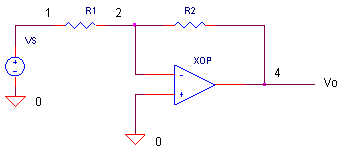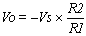eCircuit  Center

### Op Amp Inverting Amplifier

CIRCUITVOLTAGE GAIN

How does an opamp device simplify the design of an amplifier? The voltage gain for the inverting configuration is set by the ratio of the feedback resistors R1 and R2, not the internal gain of the opamp itself.Run a simulation of the SPICE circuit file “opinv.cir”. Then, plot the input V(1) and output V(4) of the Transient Analysis. With Vs=1Vp-p, R1 = 10k and R2 = 20k, does the amplifier produce an inverted 2 Vp-p at V(4)?

HANDS-ON DESIGN  You can change the gain by choosing a new value for R2. Double or triple R2 and calculate the new output voltage. (Example: Choose R2 = 40k and calculate Vo = -1 x 40k / 10k = -4.)  Edit the file with your new R2 value. Save the file and run a new simulation. Is the output at V(4) what you expected?

VIRTUAL GROUND

What is meant by the term “virtual ground”? Find out by looking at the opamp’s negative input terminal. Run a simulation and add a transient plot for V(2). You should see that the negative input V(2) is very small regardless of the input or output voltage. How small is the voltage? You could say its so small that its “virtually” at ground!

BANDWIDTH

What is the bandwidth of your amplifier? Run a simulation with R1=R2=10k and view the AC Analysis of the circuit. The flat part of the magnitude VM(4) shows the frequencies within the bandwidth that the circuit is capable of amplifying. Above the bandwidth of the amplifier, the response decreases.

CIRCUIT ANALYSIS  What happens to the bandwidth when you adjust the gain higher? Increase the gain by increasing R2 to a value like 100k. Does the bandwidth increase or decrease? Here's a a classic compromise to be reckoned with: the trade-off between gain and bandwidth.

SIMULATION NOTE

The innards of the opamp device is defined by a subcircuit. The statement XOP 0 2 4 OPAMP1 tells SPICE that the opamp device XOP is described by subcircuit XOPAMP1.The components of the device are listed between the statements .SUBCKT OPAMP1 1 2 6 and .ENDS. During the simulation, nodes (1), (2) and (6) of the subcircuit connect to nodes 0, 2 and 4 of the main circuit, respectively. (Note, the internal nodes and component names of a subcircuit are separate from those in the main circuit.) You can create a circuit with many opamps all defined by a single subcircuit. For a more detailed description of the opamp, see the Basic Opamp Model and Why Use Subcircuits?

SPICE FILE

Download the file or copy this netlist into a text file with the *.cir extention.

```OPINV.CIR - OPAMP INVERTING AMPLIFIER
*
VS	1	0	AC	1	SIN(0V	1VPEAK	10KHZ)
*
R1	1	2	5K
R2	2	4	10K
XOP	0 2	4	OPAMP1
*
* OPAMP MACRO MODEL, SINGLE-POLE
* connections:      non-inverting input
*                   |   inverting input
*                   |   |   output
*                   |   |   |
.SUBCKT OPAMP1      1   2   6
* INPUT IMPEDANCE
RIN	1	2	10MEG
* GAIN BW PRODUCT = 10MHZ
* DC GAIN (100K) AND POLE 1 (100HZ)
EGAIN	3 0	1 2	100K
RP1	3	4	1K
CP1	4	0	1.5915UF
* OUTPUT BUFFER AND RESISTANCE
EBUFFER	5 0	4 0	1
ROUT	5	6	10
.ENDS
*
* ANALYSIS
.AC 	DEC 	5 1K 10MEG
.TRAN 	0.005MS  0.2MS
* VIEW RESULTS
.PRINT	AC 	V(1) V(4)
.PRINT	TRAN 	V(1) V(4)
.PROBE
.END```

top